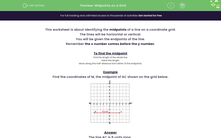# Find the Midpoint of a Line on a Grid

In this worksheet, students will find the coordinates of the midpoint of a horizontal or vertical line, given its endpoints.Key stage:  KS 3

Curriculum topic:   Algebra

Curriculum subtopic:   Use Coordinates in All Four Quadrants

Popular topics:   Algebra worksheets

Difficulty level:#### Worksheet Overview

This activity is about identifying the midpoints of a line on a coordinate grid.

The lines will be horizontal or vertical.

You will be given the endpoints of the line.

Remember the x number comes before the y number.

To find the midpoint

- Find the length of the whole line.

- Halve this length.

- Move along this half-distance from either of the endpoints.

Example

Find the coordinates of M, the midpoint of AC shown on the grid below.The line AC is 8 units long.

Count the squares to check.

A has an x-coordinate of -5.

B has an x-coordinate of +3.

From -5 up to +3 is 8 units.

The half-distance is 8 ÷ 2 = 4 units.M is the midpoint on the line AC.

It is 4 units to the right of A and 4 units to the left of C.

The coordinates of M are (-1, -3).

That wasn't too tricky, was it? Let's have a go at some questions now.

### What is EdPlace?

We're your National Curriculum aligned online education content provider helping each child succeed in English, maths and science from year 1 to GCSE. With an EdPlace account you’ll be able to track and measure progress, helping each child achieve their best. We build confidence and attainment by personalising each child’s learning at a level that suits them.

Get started